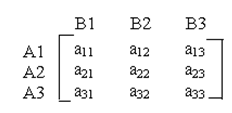#### Games with Mixed Strategies

Games with Mixed Strategies

In some cases, no pure strategy solutions present for the game. In other words, saddle point does not present. In all these games, both players may accept an optimal mix of the strategies known as Mixed Strategy to determine a saddle point. The optimal mix for each player may be determined by allocating each strategy a probability of it being selected. Therefore these mixed strategies are probabilistic combinations of accessible better strategies and these games consequently known as Probabilistic games.

The probabilistic mixed strategy games without saddle points are generally solved by any of the below specified methods

 Sl. No. Method Applicable to 1 Analytical Method 2x2 games 2 Graphical Method 2x2, mx2 and 2xn games 3 Simplex Method 2x2, mx2, 2xn and mxn games

Analytical Method

A 2 x 2 payoff matrix where there is no saddle point can be solved with the help of analytical method.

Given below the matrix

[ a11 a12  ]

a21  a22

Value of the game is

V=  (a11a22 - a21a12) / (a11+a22) - (a12+a21)

With the coordinates

With the coordinates

x1 = (a22 – a21)/(a11+a22)-(a12+a21) , x2 =    a11- a12 / (a11+a22) – (a12+a21)

y1 =  a22 - a12  / (a11+a22)-(a12+a21),  y2 =    a11- a21/ (a11+a22) – (a12+a21)

Alternative process to solve the strategy

• Find out the difference between two numbers in column 1 and enter the resulting under column 2. Ignore the negative sign if it takes place.
• Determine the difference of two numbers in column 2 and enter the resulting under column 1. Ignore the negative sign if it takes place.
• Repeat this procedure again for the two rows.

Graphical method

The graphical method is required to solve the games whose payoff matrix has

• m rows and two columns (m x 2)
• Two rows and n columns (2 x n)

Algorithm for solving 2 x n matrix games

• Make two vertical axes 1 unit apart. The two lines are as follows x1 = 0, x1 = 1
• Get the points of the Ist row in the payoff matrix on the vertical line x1 = 1 and the points of the IInd row in the payoff matrix on the vertical line x1 = 0.
• The point a1j on axis x1 = 1 is then connected to the point a2j on the axis x1 = 0 to provide a straight line. Make 'n' straight lines for j=1, 2... n and find out the highest point of the lower envelope achieved. This point will be the maximin point.
• The two or more lines passing from the maximin point provides the required 2 x 2 payoff matrix. This in turn provides the best solution by making use of analytical method.

Algorithm for solving m x 2 matrix games

• Make two vertical axes 1 unit apart. The two lines are x1 =0, x1 = 1
• Take the points of the Ist row in the payoff matrix on the vertical line x1 = 1 and the points of the IInd row in the payoff matrix on the vertical line x1 = 0.
• The point a1j on axis x1 = 1 is then connected to the point a2j on the axis x1 = 0 to provide a straight line. Make ‘n’ straight lines for j=1, 2… n and find out the lowest point of the upper envelope achieved. This point will be the minimax point.
• The two or more lines passing from the minimax point provides the required 2 x 2 payoff matrix which in turn provides the best solution by making use of analytical method.

Simplex Method

Assume the 3 x 3 matrixAccording to the assumptions, A always tries to select the set of strategies with the non-zero probabilities like p1, p2, p3 where p1 + p2 + p3 = 1 that maximizes his least expected gain.

Likewise B would select the set of strategies with the non-zero probabilities like q1, q2, q3 where q1 + q2 + q3 = 1 that minimizes his highest expected loss.

Step 1

Determine the minimax and maximin value in the given matrix

Step 2

The purpose of A is to maximize the value, which is equal to minimizing the value 1/V. The LPP can be written as

Min 1/V = p1/V + p2/V + p3/V

& constraints ≥ 1

It can be written as

Min 1/V = x1 + x2 + x3

& constraints ≥ 1

Likewise for B, we obtain the LPP as the dual of the above LPP

Max 1/V = Y1 + Y2 + Y3

& constraints ≤ 1

Where Y1 = q1/V, Y2 = q2/V, Y3 = q3/V

Step 3

Work out the LPP with the use of simplex table and get the optimum strategy for the players..